Home > GC > Chapter 6 > Lesson 6.2.5 > Problem6-84

6-84.
1. For each part below, decide if the triangles are similar. If they are similar, use their similarity to solve for x. If they are not similar, explain why not. Homework Help ✎

1.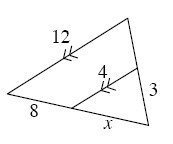2.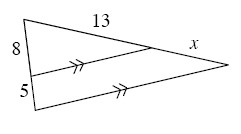3.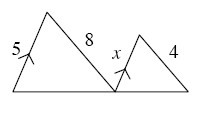4.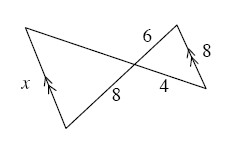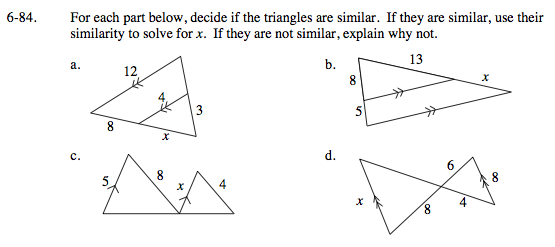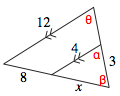Parallel lines have equal corresponding angles, so θ = α.
By shared angles, β = β.
By AA~, the triangles are similar.

$\frac{12}{4}=\frac{8+x}{x}$

12x = 4(8+x)
12x = 32 + 4x
8x = 32
x = 4

Is there enough information to prove that the triangles are similar?

Use the same method as for part (a).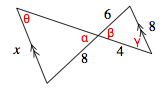Vertical angles are equal, so α = β.
Parallel lines have equal alternate interior angles,
so θ = γ.
Triangles are similar by AA~.

$\frac{6}{8}=\frac{8}{\textit{x}}$

6x = 8(8)
6x = 64
x ≈ 10.6